# Methods and formulas for the fits and residuals in Analyze Definitive Screening Design

## Fit

### Notation

TermDescription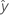fitted value
xkkth term. Each term can be a single predictor, a polynomial term, or an interaction term.
bkestimate of kth regression coefficient

## Standard error of fitted value (SE Fit)

The standard error of the fitted value in a regression model with one predictor is:

The standard error of the fitted value in a regression model with more than one predictor is:

### Notation

TermDescription
s2mean square error
nnumber of observations
x0new value of the predictor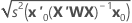mean of the predictor
xiith predictor value
x0 vector of values that produce the fitted values, one for each column in the design matrix, beginning with a 1 for the constant term
x'0transpose of the new vector of predictor values
Xdesign matrix

## Residuals

The residual is the difference between an observed value and the corresponding fitted value. This part of the observation is not explained by the model. The residual of an observation is:

### Notation

TermDescription
yiith observed response valueith fitted value for the response

## Standardized residual (Std Resid)

Standardized residuals are also called "internally Studentized residuals."

### Notation

TermDescription
ei i th residual
hi i th diagonal element of X(X'X)–1X'
s2 mean square error
Xdesign matrix
X'transpose of the design matrix

## Deleted (Studentized) residuals

Also called the externally Studentized residuals. The formula is:

Another presentation of this formula is:

The model that estimates the ith observation omits the ith observation from the data set. Therefore, the ith observation cannot influence the estimate. Each deleted residual has a student's t-distribution with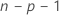degrees of freedom.

### Notation

TermDescription
eiith residual
s(i)2mean square error calculated without the ith observation
hi i th diagonal element of X(X'X)–1X'
nnumber of observations
pnumber of terms, including the constant
SSEsum of squares for error

## Confidence interval

The range in which the estimated mean response for a given set of predictor values is expected to fall.

### Notation

TermDescription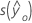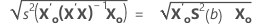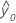fitted response value for a given set of predictor values
α type I error rate
n number of observations
p number of model parameters
S 2(b)variance-covariance matrix of the coefficients
s 2 mean square error
X design matrix
X0 vector of given predictor values
X'0transpose of the new vector of predictor values

## Prediction interval

The prediction interval is the range in which the fitted response for a new observation is expected to fall.

### Notation

TermDescription
s(Pred)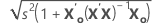fitted response value for a given set of predictor values
α level of significance
n number of observations
p number of model parameters
s 2 mean square error
X predictor matrix
X0 matrix of given predictor values
X'0transpose of the new vector of predictor values
By using this site you agree to the use of cookies for analytics and personalized content.  Read our policy